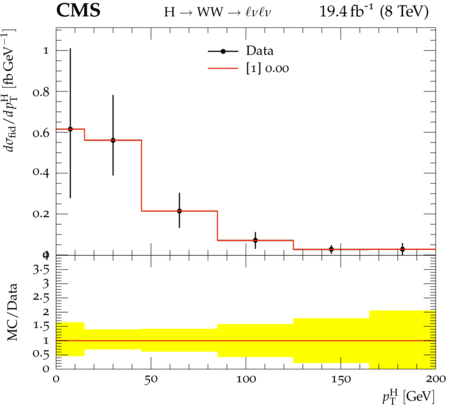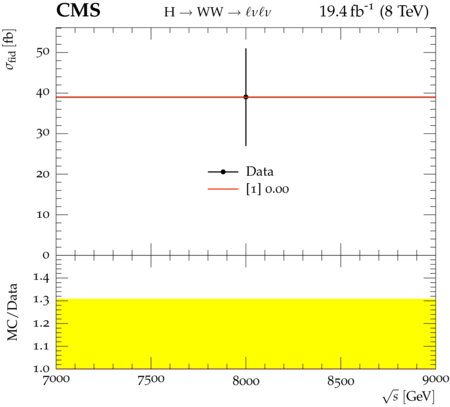### CMS_2017_I1467451

Back to index

The cross section for Higgs boson production in pp collisions is studied using the $H\rightarrow W^+ W^-$ decay mode, followed by leptonic decays of the W bosons to an oppositely charged electron-muon pair in the final state. The measurements are performed using data collected by the CMS experiment at the LHC at a centre-of-mass energy of 8 TeV, corresponding to an integrated luminosity of $19.4\,\text{fb}^{-1}$. The Higgs boson transverse momentum ($p_\text{T}$) is reconstructed using the lepton pair $p_\text{T}$ and missing $p_\text{T}$. The differential cross section times branching fraction is measured as a function of the Higgs boson $p_\text{T}$ in a fiducial phase space defined to match the experimental acceptance in terms of the lepton kinematics and event topology. The production cross section times branching fraction in the fiducial phase space is measured to be $39 \pm 8\,\text{(stat)} \pm 9\,\text{(syst)}\,\text{fb}$. The measurements are found to agree, within experimental uncertainties, with theoretical calculations based on the standard model. The fiducial region is defined at particle level (using Born level leptons) as: - emununu, leptons are required not to originate from leptonic tau decays - leading lepton $p_T > 20$ GeV - subleading lepton $p_T > 10$ GeV - psudorapidity of electrons and muons $|\eta| < 2.5$ - Invariant mass of the two charged leptons $m_{ll} > 12$ GeV - Charged lepton pair $p_T > 30$ GeV - Invariant mass of the leptonic system in the transverse plane $m_T^{e\mu\nu\nu} > 50$ GeV

CMS_2017_I1467451_d01-x01-y01:CMS_2017_I1467451_d02-x01-y01:Generated at Sunday, 28. January 2018 12:08PM